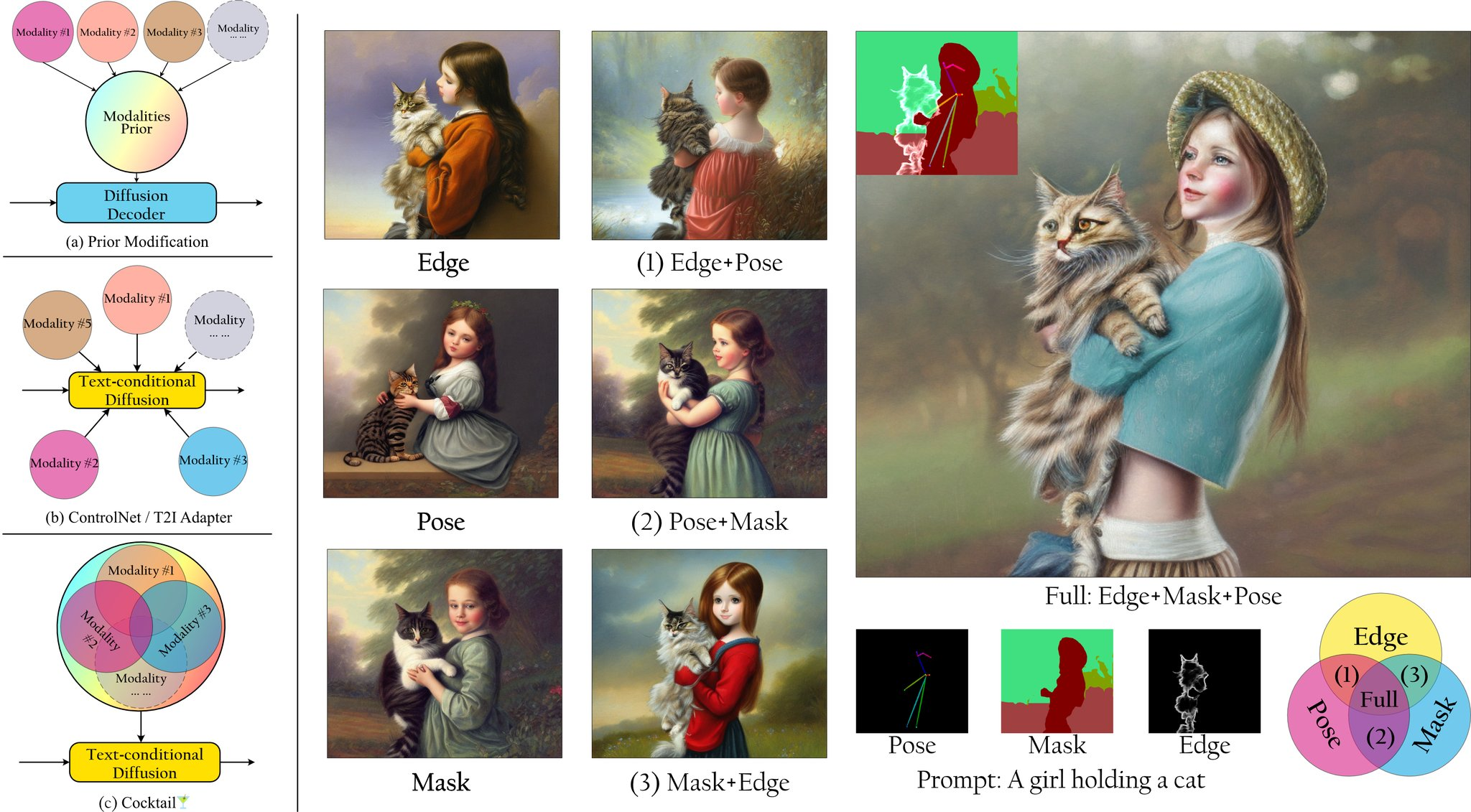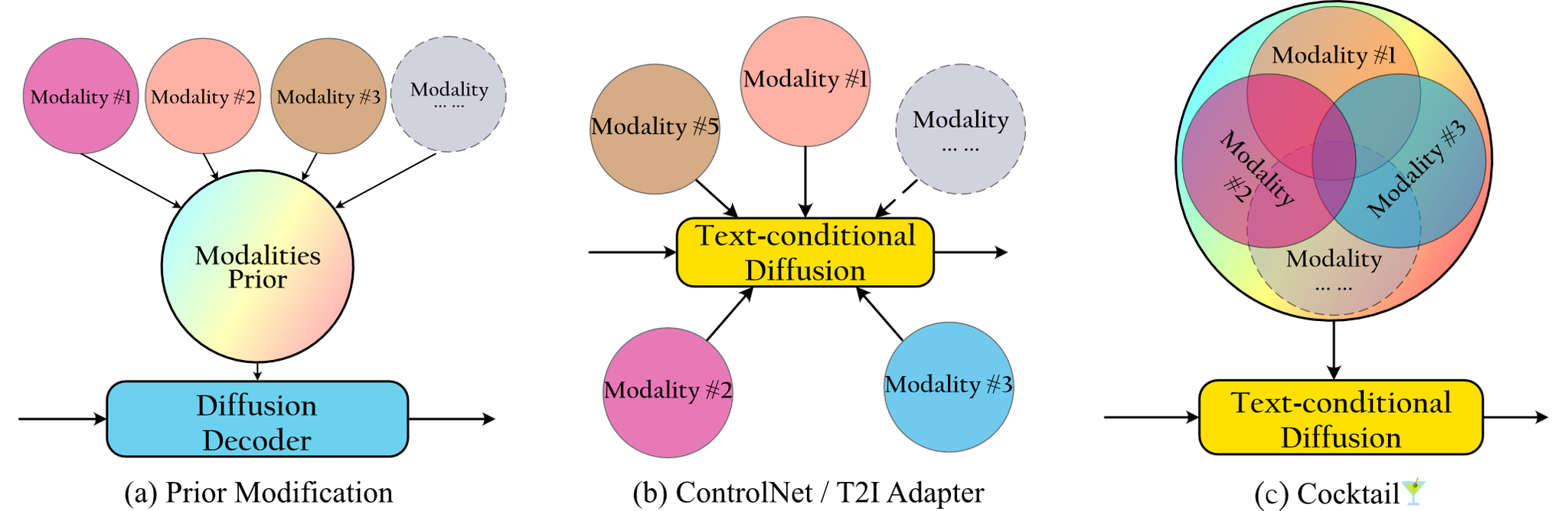# Cocktail🍸: Mixing Multi-Modality Controls for Text-Conditional Image Generation

##### Video## Abstract

Text-conditional diffusion models are able to generate high-fidelity images with diverse contents.However, linguistic representations frequently exhibit ambiguous descriptions of the envisioned objective imagery, requiring the incorporation of additional control signals to bolster the efficacy of text-guided diffusion models. In this work, we propose Cocktail, a pipeline to mix various modalities into one embedding, amalgamated with a generalized ControlNet (gControlNet), a controllable normalisation (ControlNorm), and a spatial guidance sampling method, to actualize multi-modal and spatially-refined control for text-conditional diffusion models. Specifically, we introduce a hyper-network gControlNet, dedicated to the alignment and infusion of the control signals from disparate modalities into the pre-trained diffusion model. gControlNet is capable of accepting flexible modality signals, encompassing the simultaneous reception of any combination of modality signals, or the supplementary fusion of multiple modality signals. The control signals are then fused and injected into the backbone model according to our proposed ControlNorm. Furthermore, our advanced spatial guidance sampling methodology proficiently incorporates the control signal into the designated region, thereby circumventing the manifestation of undesired objects within the generated image. We demonstrate the results of our method in controlling various modalities, proving high-quality synthesis and fidelity to multiple external signals.## Overall Pipeline

Our approach requires only one generalized model, unlike previous that needed multiple models for multiple modalities. Different from currently existing schemes, our scheme does not require modifications to the modal prior of the base model Fig.(a), which results in a significant reduction in cost. Also in the face of multiple modalities we do not need multiple models demonstrated in Fig.(b). Cocktail🍸 fuse the information from multiple modalities like Fig.(c) shown.The noise-perturbed images are injected into the gControlNet via the ControlNorm, which also channels the dimensionally distinct control signals obtained from the gControlNet into the pre-trained network with rich semantic information.## Generalized ControlNet

Given a trained backbone network block $$\mathcal{F}(\cdot;\boldsymbol{\theta})$$ with parameter$$\boldsymbol{\theta}$$, the input feature$$\boldsymbol{x}$$ can be mapped to $$\boldsymbol{y}$$. For the branched part, we duplicate the parameter $$\boldsymbol{\theta}$$ to create a trainable copy $$\boldsymbol{\theta}_t$$, which is then trained using the supplementary modality. Preserving the original weights helps retain the information stored in the initial model after training on large-scale datasets, which ensures that the quality and diversity of the generated images do not degrade. Mathematically, the output from the trained network block can be expressed as:

$\boldsymbol{y} = \mathcal{F}(\boldsymbol{x}; \boldsymbol{\theta}) + \mathcal{Z}\left(\mathcal{F}(\boldsymbol{x}+\mathcal{Z}(\boldsymbol{c}_m) ; \boldsymbol{\theta}_t)\right) \leftarrow \mathcal{F} (\boldsymbol{x} ; \boldsymbol{\theta}) ,$

To accomplish the goals of accepting multiple external modalities as input and balancing signals from different modalities, we have devised a modified framework that adeptly merges these varied sources of information. At the top of our network, we adopt a simple downsampling network $$\mathcal{M}(\cdot)$$ to convert external conditional signals to the latent space, allowing the conditional signals to be directly injected into the latent space. It is worth noting that $$\mathcal{M}(\cdot)$$ is versatile and can adapt to different types of external signals. Given $$k$$ different modalities, the converted conditional features are $$\boldsymbol{c}_m ^ k = \mathcal{M} (C ^ k)$$.

## Controllable Normalisation

Instead of directly passing the sum of conditional features via a zero-initialized layer to the network block $$\mathcal{F}(\cdot;\boldsymbol{\theta}_t)$$, i.e., $$\hat{\boldsymbol{c}}_m = \mathcal{Z}(\sum_i\boldsymbol{c}^i)$$, we instead introduce a controllable normalisation (ControlNorm), which has an additional layer to generate two sets of learnable parameters, $$\boldsymbol {\gamma}(\hat{\boldsymbol{c}}_m)$$ and $$\boldsymbol {\beta}(\hat{\boldsymbol{c}}_m)$$, conditioned on all$$k$$ modalities. These two sets of parameters are used in the conditional normalisation layer to fuse the external conditional signals and the original signals.

$\left(\boldsymbol {I}+\mathcal{Z}(\boldsymbol {\gamma}\left(\hat{\boldsymbol{c}}_m\right))\right) \odot \frac{\boldsymbol{x} - \mu_c(\boldsymbol{x})}{\sigma_c(\boldsymbol{x})} \oplus \mathcal{Z}(\boldsymbol {\beta}(\hat{\boldsymbol{c}}_m)) \leftarrow \boldsymbol{x} +\mathcal{Z}(\boldsymbol{c}_m),$

In fact, our controllable normalisation is a generalized version of conditional normalisation. After changing the mean and variance calculation dimension and replacing the external signal by a mask image, real image, or class labels, we can derive the various forms of SPADE, AdaIN, CIN and MoVQ. More interestingly, our controllable normalisation method not only enables the use of external signals as conditions, but also allows intermediate layer signals to act as constraints.

Our proposed gControlNet shares the same objective function as the diffusion model, aiming to predict the noise added at time t. The only distinction lies in the incorporation of multimodal information:

$\mathcal{L} = \mathbb{E}_{\boldsymbol{z}_{0}, t, \boldsymbol{c}_p, \hat{\boldsymbol{c}}_m, \epsilon \sim \mathcal{N}(0,1)} \left[ \Vert \epsilon - \epsilon_{\theta}\left(\boldsymbol{z}_t, t, \boldsymbol{c}_p,\hat{\boldsymbol{c}}_m \right) \Vert ^2_2 \right]$

## Spatial Guidance Sampling

We apply a masking strategy to the corresponding attention maps. In detail, we construct two sets of attention masks $$M^{\text{pos}(n)}$$ and $$M^{\text{neg}(n)} \in \mathbb{R}^{(N_i,N_t)}$$. Each column $$M^{\text{pos}(n)}_j$$ and $$M^{\text{neg}(n)}_j$$ is a flattened alpha mask, which is determined by the visibility of the corresponding text token $$K_j$$. The values of $$M^{\text{pos}(n)}_{ij}$$ and $$M^{\text{neg}(n)}_{ij}$$ are determined based on the relationship between image token $$Q_i$$ and text token $$K_j$$. Specifically, if image token $$Q_i$$ corresponds to a region of the image that should be influenced by text token $$K_j$$, $$M^{\text{pos}(n)}_{ij}$$ is assigned the value of 1. On the other hand, if image token $$Q_i$$ corresponds to a region of the image that should not be influenced by text token $$K_j$$, $$M^{\text{neg}(n)}_{ij}$$ is set to 1. The mask components $$M^{\text{pos}(n)}$$ and $$M^{\text{neg}(n)}$$ are incorporated into the cross-attention computation process: $\tilde{A}^{(n)}_{ij|\boldsymbol{\theta}^{(n)}} = \frac{\text{exp}\langle Q^{(n)}_i,K_j\rangle + \omega^{\text{pos}} M^{\text{pos}(n)} - \omega^{\text{neg}} M^{\text{neg}(n)} }{\sum_{k=1} \text{exp} \langle Q^{(n)}_i,K_k \rangle}.$

## Experimental Results

### Multi-Modality Generation

Cocktail🍸 is proficient in seamlessly supporting multiple control inputs and autonomously fusing them, thereby eliminating the necessity for manual intervention to equilibrate diverse modalities. This unique property empowers users to easily incorporate a variety of modalities, resulting in more flexible multi-modal control.### Multi-Modality Comparision

Our proposed Cocktail🍸 can generate a structural image that closely resembles the ground truth image and aligns better with the input conditions, establishing its superiority.###### Cocktail🍸 can address the imbalance among various modalities.### Uni-Modality Comparision

Our method also performs well on uni-modality translation.### Quantitative Evaluation

Methods Similarity / LPIPS ↓ Sketch Map / L2 ↓ Segmentation / mPA ↑ Segmentation / mIoU ↑ Pose Map / mAP ↑
Multi-ControlNet 0.66527 ± 0.00145 7.59721 ± 0.01516 0.36592 ± 0.00273 0.22696 ± 0.00229 0.38189 ± 0.00761
Multi-Adapter 0.72716 ± 0.00120 7.93310 ± 0.01392 0.26304 ± 0.00242 0.13981 ± 0.00177 0.40018 ± 0.00761
Ours w/o ControlNorm 0.48999 ± 0.00141 7.18413 ± 0.01453 0.48263 ± 0.00287 0.32661 ± 0.00272 0.61931 ± 0.00775
Cocktail🍸 0.48357 ± 0.00133 7.28929 ± 0.01385 0.49203 ± 0.00289 0.33267 ± 0.00271 0.61990 ± 0.00778

## Citation

 @article{hu2023cocktail,   title = {Cocktail: Mixing Multi-Modality Controls for Text-Conditional Image Generation},   author = {Hu, Minghui and Zheng, Jianbin and Liu, Daqing and Zheng, Chuanxia and Wang, Chaoyue and Tao, Dacheng and Cham, Tat-Jen},   journal = {arXiv},   year = {2023}, }

### Acknowledgements

The website template was borrowed from DreamFusion.